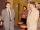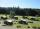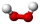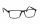# Cacao

Cacao contains 34% filling. How many grams of filling are in 130 g cacao.

Correct result:

x =  44.2 g

#### Solution:We would be pleased if you find an error in the word problem, spelling mistakes, or inaccuracies and send it to us. Thank you!Tips to related online calculators

## Next similar math problems:

• GramsHow many grams of 5% solution do we need to add to 100g of 50% solution to get a 20% solution?
• Acid solutionBy adding 250 grams of a 96% solution of sulfuric acid to its 3% solution its initial concentration was changed to 25%. How many grams of 3% of the acid were used for dilution?
• Percents - easyHow many percent is 432 out of 434?
• Base of percentages7% of the base is 35 kg. What is 39% of that base?
• Two workersTwo workers make 138 parts/items. The first one produces 30% more than the second. How many items will each produce?
• Acid evaporationHow many kilograms of water do we have to evaporate from 100 kg of 32% acid to make it 80% concentration?
• PeroxideHow many ml 30% of peroxide (H2O2) should be poured into 100ml H2O to give a 20% solution?
• Perctentages35% of what number is 35?
• ResortIn a completely crowded resort was 30% from the Czech Republic quarter visitors were from Slovakia and the rest 135 came from Germany. What capacity have resort?
• SolutionsHow much 60% solution and how much 35% solution is needed to create 100 l of 40% solution?
• CloggingHow much distilled water must the pharmacist add to 30g of a 30% hydrogen peroxide solution to obtain a 3% solution to clogging?
• GardenThe garden around the new farm is divided as follows: 35% vegetable, 30% fruit orchards, 10% flowers and the remaining 120 m2 are lawns. What is the total area of the garden?
• CuSO4 mixtureHow many grams of solid CuSO4 we have to add to 450g of 15% CuSO4 solution to produce a 25% solution?
• Percentages above 100%What is 122% of 185? What is the meaning of percentages above 100%?
• WeightlifterWeightlifter lifted 75% of its weight. Determine how much weight lifted when he weighs 132 kg.
• In the cinemaThere were 560 people in the cinema. 35% of them wore glasses. Of this number, 25% were women. How many men wore glasses?
• ConcentrationHow many grams of 65% and 50% acid is to be mixed to give 240 g acid with a concentration of 60%?